# 表批读写

Delta Lake支持Apache Spark DataFrame读写API提供的大多数选项，用于对表执行批量读写。

## 建立表格

Delta Lake支持使用DataFrameWriter（Scala/Java / Python）直接基于路径创建表。Delta Lake还支持使用标准DDL CREATE TABLE在元存储中创建表。 使用Delta Lake在元存储中创建表时，它将表数据的位置存储在元存储中。此方式使其他用户更容易发现和引用数据，而无需担心数据存储的准确位置。但是，元存储不是表中有效内容的真实来源。数据内容仍然由Delta Lake负责存储。

SQL

``````%sql
-- Create table in the metastore
CREATE TABLE events (
date DATE,
eventId STRING,
eventType STRING,
data STRING)
USING DELTA``````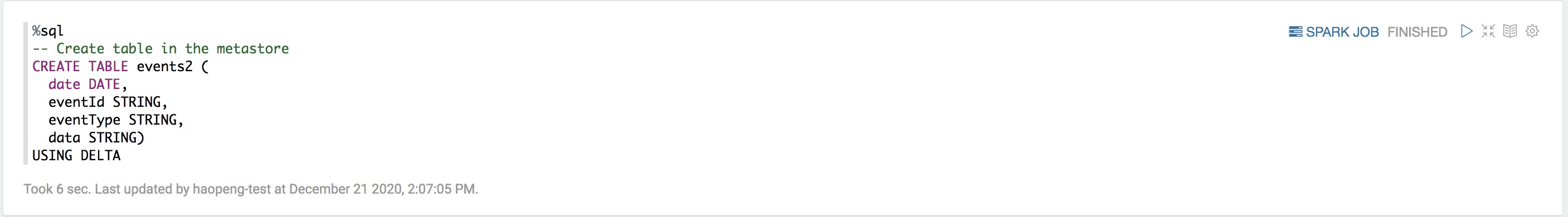Python

``````%pyspark
df = spark.createDataFrame([("case21", '2020-10-12', 21, 'INFO'),("case22", '2020-10-13', 22, 'INFO')], ['data', 'date', 'eventId', 'eventType'])
df.write.format("delta").saveAsTable("events2")      # create table in the metastore

df.write.format("delta").save("/mnt/delta/events3")  # create table by path``````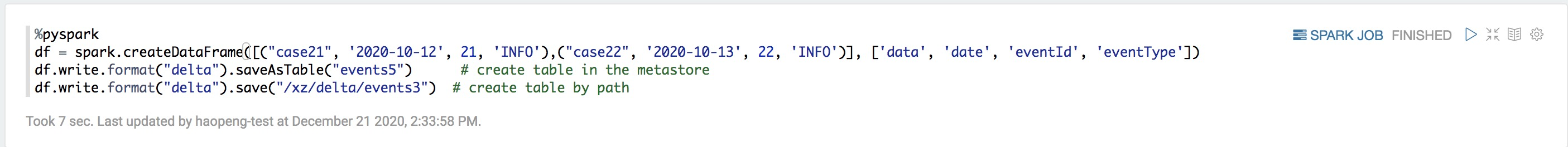Scala

``````%spark
val df = spark.createDataFrame(Seq(("case21", "2020-10-12", 21, "INFO"))).toDF("data", "date", "eventId", "eventType")
df.write.format("delta").saveAsTable("events4")      // create table in the metastore

df.write.format("delta").save("/mnt/delta/events5")  // create table by path``````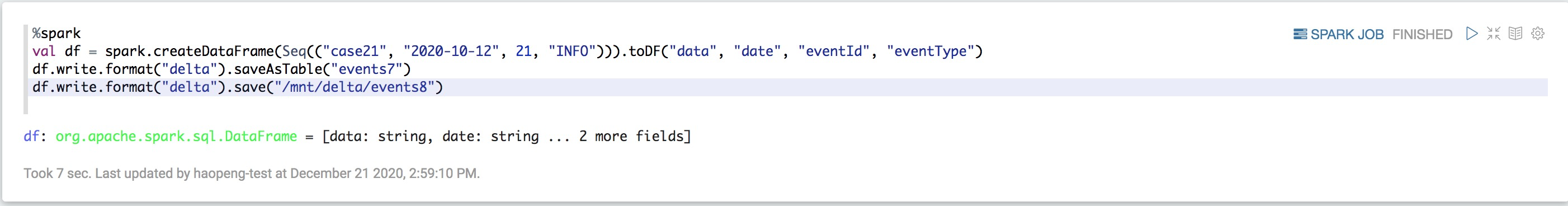SQL

``````%sql
-- Create a table by path
CREATE OR REPLACE TABLE delta.`/mnt/delta/events` (
date DATE,
eventId STRING,
eventType STRING,
data STRING)
USING DELTA
PARTITIONED BY (date);

-- Create a table in the metastore
CREATE OR REPLACE TABLE events (
date DATE,
eventId STRING,
eventType STRING,
data STRING)
USING DELTA
PARTITIONED BY (date);``````

SCALA

``````%spark
val df = spark.createDataFrame(Seq(("case21", "2020-10-12", 21, "INFO"))).toDF("data", "date", "eventId", "eventType")
df.writeTo("delta.`/mnt/delta/events`").using("delta").partitionedBy("date").createOrReplace() // create table by path

df.writeTo("events").using("delta").partitionedBy("date").createOrReplace()                   // create table in the metastore``````

## 分区数据

SQL

``````%sql
-- Create table in the metastore
CREATE TABLE events (
date DATE,
eventId STRING,
eventType STRING,
data STRING)
USING DELTA
PARTITIONED BY (date)
LOCATION '/mnt/delta/events'``````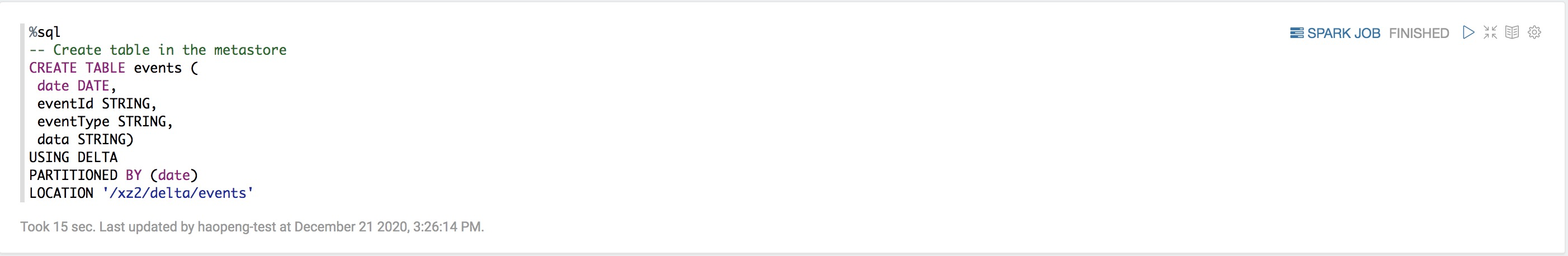Python

``````%pyspark
df = spark.createDataFrame([("case21", '2020-10-12', 21, 'INFO'),("case22", '2020-10-13', 22, 'INFO')], ['data', 'date', 'eventId', 'eventType'])
df.write.format("delta").partitionBy("date").saveAsTable("events1")      # create table in the metastore

df.write.format("delta").partitionBy("date").save("/mnt/delta/events2")  # create table by path``````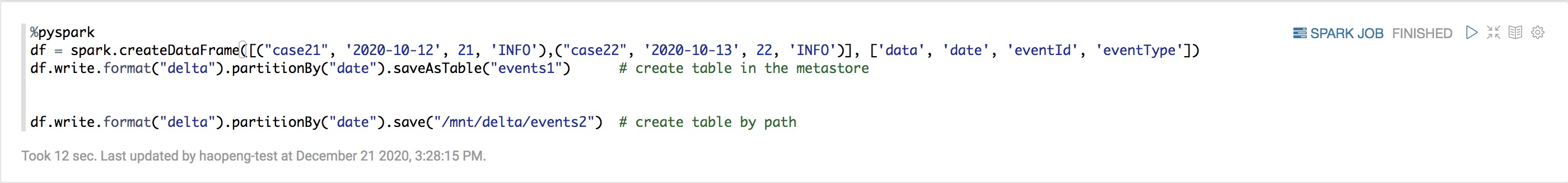Scala

``````%spark
val df = spark.createDataFrame(Seq(("case21", "2020-10-12", 21, "INFO"))).toDF("data", "date", "eventId", "eventType")
df.write.format("delta").partitionBy("date").saveAsTable("events3")      // create table in the metastore

df.write.format("delta").partitionBy("date").save("/mnt/delta/events4")  // create table by path``````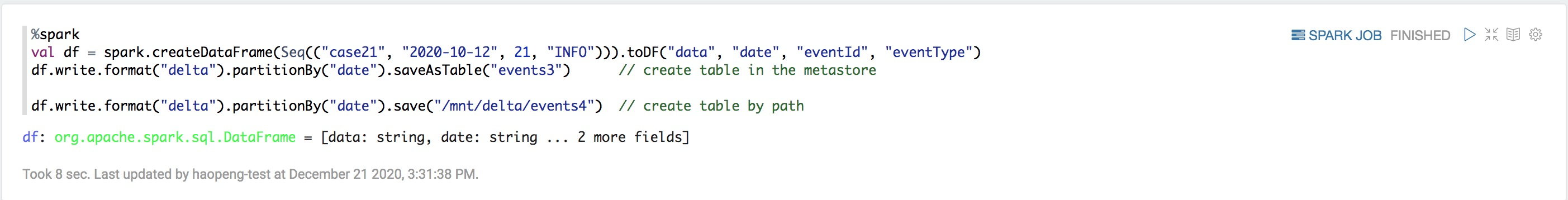## 控制数据位置

SQL

``````%sql
CREATE TABLE events
USING DELTA
LOCATION '/mnt/delta/events'``````

Hive Metastore中的表会自动继承现有数据的Schema，分区和表属性。此功能可用于将数据“导入”到元存储中。

## 读取表

SQL

``````%sql
SELECT * FROM events   -- query table in the metastore

SELECT * FROM delta.`/mnt/delta/events`  -- query table by path``````

Python

``````%pyspark
spark.table("events")    # query table in the metastore

Scala

``````%spark
spark.table("events")      // query table in the metastore

## 查询表的旧快照（按时间顺序查看）

Delta Lake按时间顺序查看允许您查询Delta表的旧快照。按时间顺序查看有很多用例，包括：

• 重新创建分析，报告或输出（例如，机器学习模型的输出）。这对于排查问题或者审计尤其有用，特别是在管控行业中。

• 编写复杂的时间查询。

• 修正数据中的错误。

• 在快速变更的表中，提供一系列的快照查询功能。

SQL AS OF 语法

``````%sql
SELECT * FROM
events
TIMESTAMP AS OF timestamp_expression
SELECT * FROM events VERSION AS OF version``````

timestamp_expression为实际的时间，你可以通过DESCRIBE HISTORY events查看表的历史版本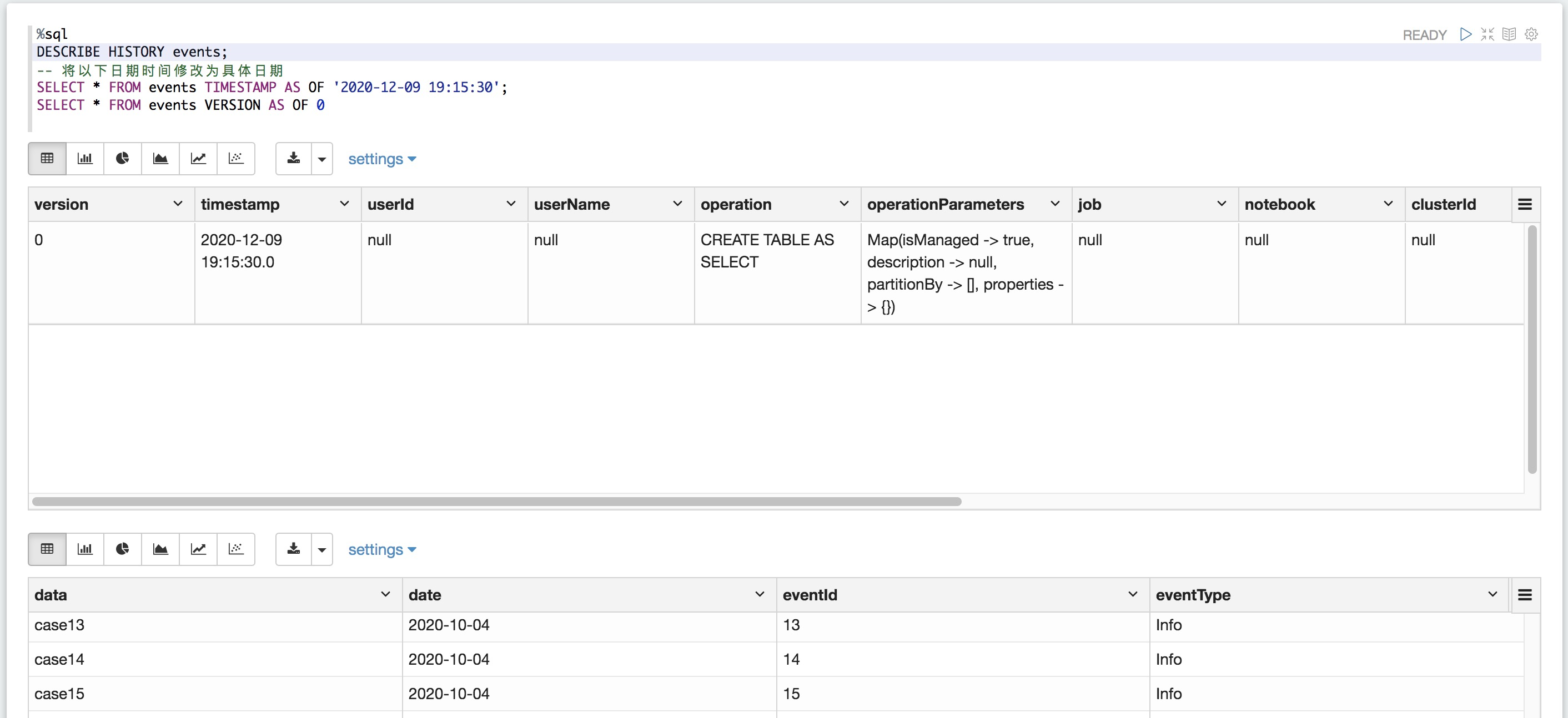table_identifier

• [database_name.] table_name：一个表名，可以选择用数据库名限定。

• delta.`<path-to-table>` ：现有Delta表的位置。

1. '2018-10-18T22:15:12.013z',即可以转换为时间戳的字符串

2. cast('2018-10-18 13:36:32 CEST' as timestamp)

3. '2018-10-18'，即日期字符串

4. 在Databricks运行时6.6及更高版本中：

1. current_timestamp() - interval 12 hours

2. date_sub(current_date(), 1)

3. 任意可以转换为时间戳的其它表达式

version是一个长整型数值，可以从DESCRIBE HISTORY table_spec查询中获取到。

SQL

``````%sql
SELECT * FROM events TIMESTAMP AS OF '2018-10-18T22:15:12.013Z'
SELECT * FROM delta.`/mnt/delta/events` VERSION AS OF 123``````

Python

``````%pyspark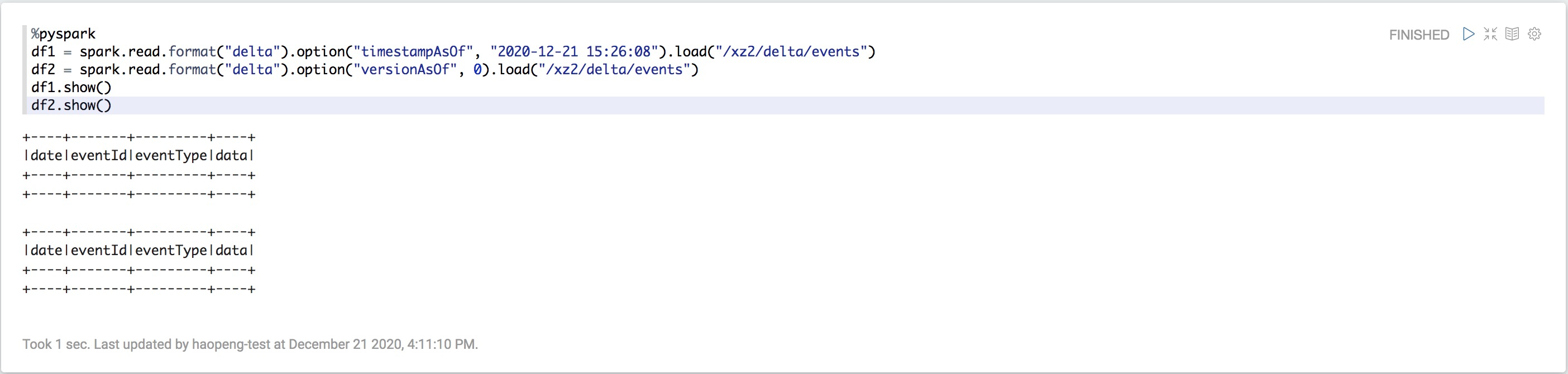Python

``````%pyspark
latest_version = spark.sql("SELECT max(version) FROM (DESCRIBE HISTORY delta.`/mnt/delta/events`)").collect()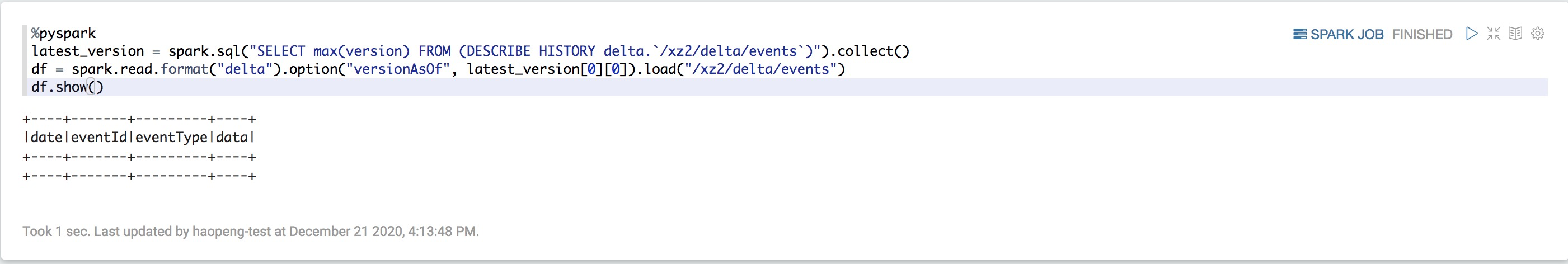SQL

``````%sql
SELECT * FROM events@20190101000000000;
SELECT * FROM events@v123``````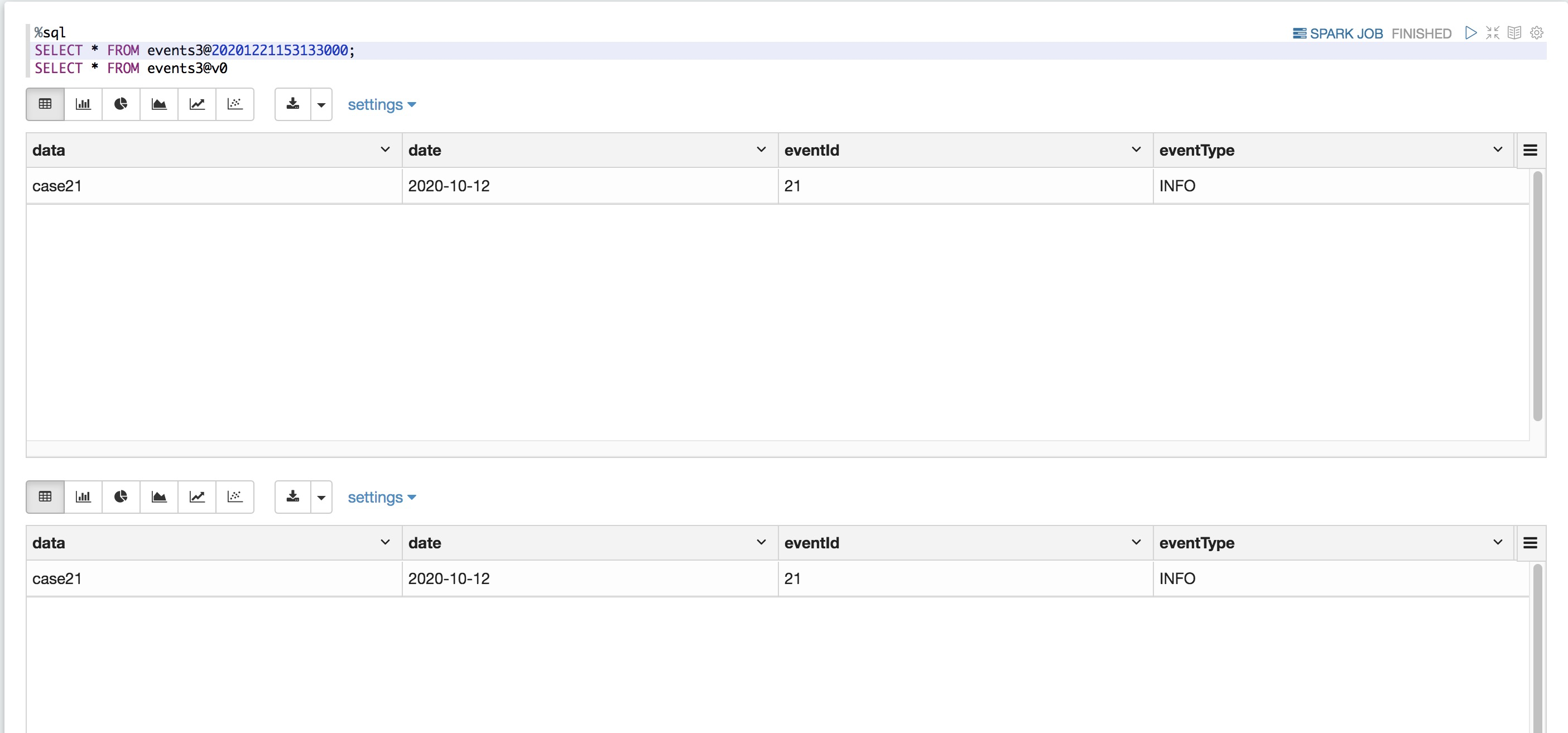Python

``````%pyspark

## 数据保存

• 您没有在Delta表上运行VACUUM。如果运行VACUUM，您将无法恢复到默认的7天数据保留期之前的版本。

• delta.logRetentionDuration = "interval <interval>"：控制表的历史记录保留时间长度。每次写入一个检查点时，Databricks都会自动清除早于保留间隔的日志条目。如果将此配置设置为足够大的值，则会保留许多日志条目。这不会影响性能，因为针对日志的操作恒定时间。历史记录的操作是并行的（但是随着日志大小的增加，它将变得更加昂贵）。默认值为interval 30 days

• delta.deletedFileRetentionDuration = "interval <interval>"：控制选择的文件必须选择时间段，默认值为间隔7天。若要访问 30 天的历史数据，请设置 delta.deletedFileRetentionDuration = "interval 30 days"。此设置可能会导致您的存储成本上升。

重要

VACUUM 不清理日志文件；写入检查点后，日志文件将自动清除。

按时间顺序查看到以前的版本，必须保留日志文件和该版本的数据文件。

## 案例

• 修复用户111表的意外删除问题：

SQL

``````%sql
INSERT INTO my_table
SELECT * FROM my_table TIMESTAMP AS OF date_sub(current_date(), 1)
WHERE userId = 111``````

• 修复对表的意外错误更新：

SQL

``````%sql
MERGE INTO my_table target
USING my_table TIMESTAMP AS OF date_sub(current_date(), 1) source
ON source.userId = target.userId
WHEN MATCHED THEN UPDATE SET *``````

• 查询过去一周增加的新客户数量。

SQL

``````%sql
SELECT count(distinct userId) - (
SELECT count(distinct userId)
FROM my_table TIMESTAMP AS OF date_sub(current_date(), 7))``````

## 写入表格

SQL

``````%sql
INSERT INTO events SELECT * FROM newEvents``````

Python

``````%pyspark
df.write.format("delta").mode("append").save("/mnt/delta/events")
df.write.format("delta").mode("append").saveAsTable("events")``````

Scala

``````%spark
df.write
.format("delta")
.mode("overwrite")
.option("replaceWhere", "date >= '2017-01-01' AND date <= '2017-01-31'")
.save("/mnt/delta/events")``````

SQL

``````%sql
INSERT OVERWRITE TABLE events SELECT * FROM newEvents``````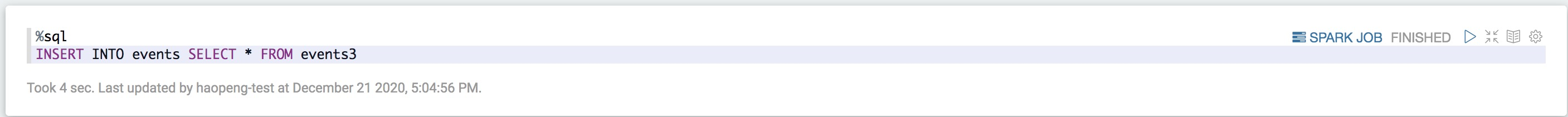Python

``````%pyspark
from pyspark.sql.functions import  to_date
df = spark.createDataFrame([("case21", '2020-10-12', 23, 'INFO'),("case22", '2020-10-13', 24, 'INFO')], ['data', 'date', 'eventId', 'eventType'])
df1 = df.select('data', to_date('date', 'yyyy-MM-dd').alias('date'), 'eventId', 'eventType')
df1.write.format("delta").mode("append").save("/mnt/delta/events")
df1.write.format("delta").mode("append").saveAsTable("events")``````

Scala

``````%spark
df.write
.format("delta")
.mode("overwrite")
.option("replaceWhere", "date >= '2017-01-01' AND date <= '2017-01-31'")
.save("/mnt/delta/events")``````

Python

``````%pyspark
df = spark.createDataFrame([("case21", '2020-10-12', 21, 'INFO'),("case22", '2020-10-13', 22, 'INFO')], ['data', 'date', 'eventId', 'eventType'])
df1 = df.select('data', to_date('date', 'yyyy-MM-dd').alias('date'), 'eventId', 'eventType')
# 可以替换分区部分数据（replaceWhere中字段必须是分区字段）;此处替换大于等于10-4的数据，并将10-12、10-13号的数据填入
df1.write.format("delta").mode("overwrite").option("replaceWhere", "date >= '2020-10-4'").saveAsTable("events_partition")
spark.table("events_partition").show(50)``````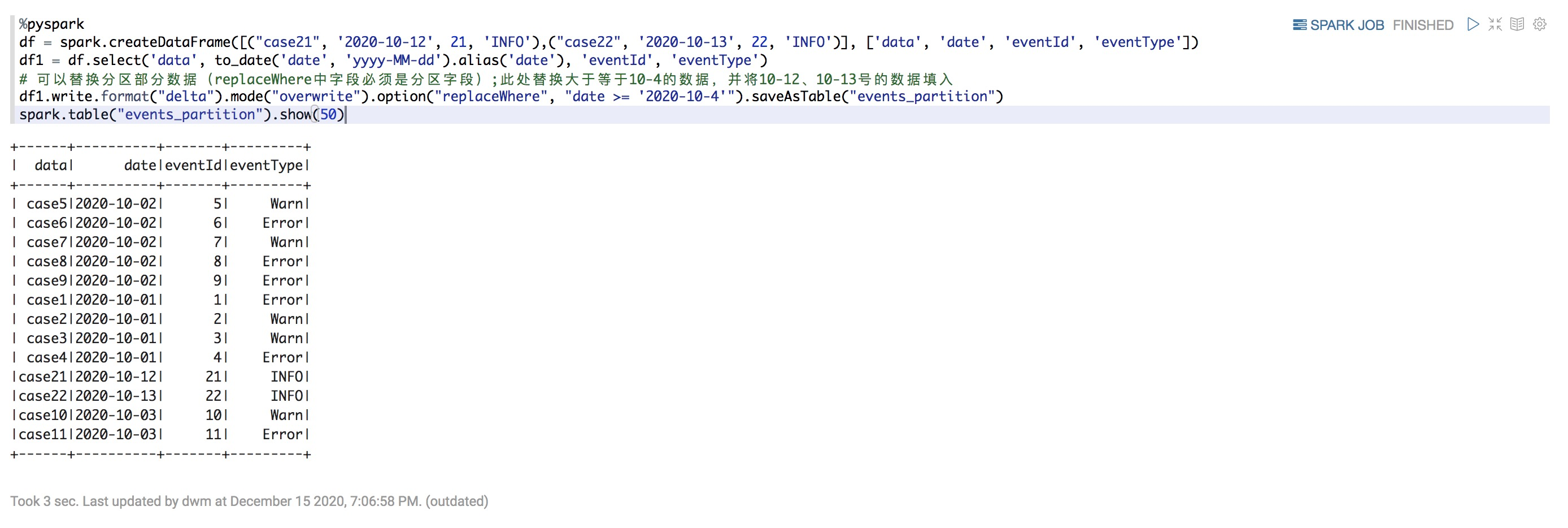Scala

``````%spark
df.write
.format("delta")
.mode("overwrite")
.option("replaceWhere", "date >= '2017-01-01' AND date <= '2017-01-31'")
.save("/mnt/delta/events")``````

## 设置用户定义的提交元数据

SQL

``````%sql
INSERT OVERWRITE events SELECT * FROM newEvents``````

Python

``````%python
df.write.format("delta") \
.mode("overwrite") \
.save("/mnt/delta/events")``````

Scala

``````%spark
df.write.format("delta")
.mode("overwrite")
.save("/mnt/delta/events")``````

## Schema验证

Delta Lake自动验证正在写入的DataFrame的Schema与表的Schema兼容。Delta Lake使用以下规则来确定从DataFrame到表的写入是否兼容：

• 所有DataFrame列都必须存在于目标表中。如DataFrame中有表中不存在列，则会抛出异常。表中存在但DataFrame中不存在的列设置为NULL。

• DataFrame列数据类型必须与目标表中的列数据类型匹配。如果它们不匹配，则会抛出异常。

• DataFrame列名称不能仅通过大小写来区分。这意味着您不能在同一表中定义诸如“ Foo”和“ foo”之类的列。虽然可以在区分大小写或不区分大小写（默认）模式下使用Spark，但在存储和返回列信息时，Parquet区分大小写。在存储Schema时，Delta Lake 保留但不区分大小写，并采用此限制来避免潜在的错误、数据损坏或丢失问题。

Delta Lake支持DDL显式添加新列，并具有自动更新Schema的功能。

## 更新表Schema

Delta Lake可让您更新表的Schema。支持以下类型的更改：

• 添加新列（在任意位置）

• 重新排序现有列

## 添加列

SQL

``````%sql
ALTER TABLE table_name ADD COLUMNS (col_name data_type [COMMENT col_comment] [FIRST|AFTER colA_name], ...)``````

SQL

``````%sql
ALTER TABLE table_name ADD COLUMNS (col_name.nested_col_name data_type [COMMENT col_comment] [FIRST|AFTER colA_name], ...)``````

``````| - colA
| - colB
| +-field1
| +-field2``````

``````| - colA
| - colB
| +-field1
| +-nested
| +-field2``````

SQL

``````%sql
ALTER TABLE table_name CHANGE [COLUMN] col_name col_name data_type [COMMENT col_comment] [FIRST|AFTER colA_name]``````

SQL

``````%sql
ALTER TABLE table_name CHANGE [COLUMN] col_name.nested_col_name nested_col_name data_type [COMMENT col_comment] [FIRST|AFTER colA_name]``````

``````| - colA
| - colB
| +-field1
| +-field2``````

``````| - colA
| - colB
| +-field2
| +-field1``````

SQL

``````%sql
ALTER TABLE table_name REPLACE COLUMNS (col_name1 col_type1 [COMMENT col_comment1], ...)``````

SQL

``````%sql
ALTER TABLE boxes REPLACE COLUMNS (colC STRING, colB STRUCT<field2:STRING, nested:STRING, field1:STRING>, colA STRING)``````

``````| - colA
| - colB
| +-field1
| +-field2``````

``````| - colC
| - colB
| +-field2
| +-nested
| +-field1
| - colA``````

Python

``````%pyspark
.withColumn("date", col("date").cast("date"))
.write
.format("delta")
.mode("overwrite")
.option("overwriteSchema", "true")
.saveAsTable(...)``````

Python

``````%pyspark
.withColumnRenamed("date", "date_created")
.write
.format("delta")
.mode("overwrite")
.option("overwriteSchema", "true")
.saveAsTable(...)``````

## 自动Schema更新

Delta Lake可以作为DML事务的一部分（附加或覆盖）自动更新表的Schema，并使该Schema与正在写入的数据兼容。

• write或writeStream有.option("mergeSchema", "true")

• spark.databricks.delta.schema.autoMerge.enabled是 true

• 当启用了表访问控制时，mergeSchema不受支持（因为它将需要MODIFY的请求为ALL PRIVILEGES的请求） 。

• mergeSchema 不能与INSERT INTO或.write.insertInto()一起使用。

NullType 列

Nulltype不支持流式传输。由于必须在使用流式传输时设置Schema，因此这种情况很少见。Nulltype也不适用于ArrayType和MapType的复杂类型。

## 替换表Schema

Python

``````%pyspark
df.write.option("overwriteSchema", "true")``````

## 表属性

TBLPROPERTIES作为Delta表元数据一部分存储。如果给定位置中已经存在Delta表，则不能在CREATE语句中定义新的TBLPROPERTIES 。

• 阻止Delta表中删除和更新：delta.appendOnly=true。

• 配置按时间顺序查看保留属性：delta.logRetentionDuration=<interval-string>和delta.deletedFileRetentionDuration=<interval-string>。

• 这些是唯一受支持的delta.前缀表属性。

• 修改Delta表属性是一种写操作，它将与其他并发的写操作发生冲突，从而导致它们失败。我们建议您仅在表上没有并发写操作时才修改表属性。

SQL

``````%sql
spark.sql("SET spark.databricks.delta.properties.defaults.appendOnly = true")``````

Scala

``````%spark
spark.conf.set("spark.databricks.delta.properties.defaults.appendOnly", "true")``````

Python

``````%pyspark
spark.conf.set("spark.databricks.delta.properties.defaults.appendOnly", "true")``````

## 元数据表

Delta Lake具有丰富的功能，可用于浏览元数据表。

• Databricks Runtime 7.0及更高版本

• Databricks Runtime 6.x及以下版本

• DESCRIBE DETAlL

• DESCRIBE HISTORY

DESCRIBE DETAlL

DESCRIBE HISTORY